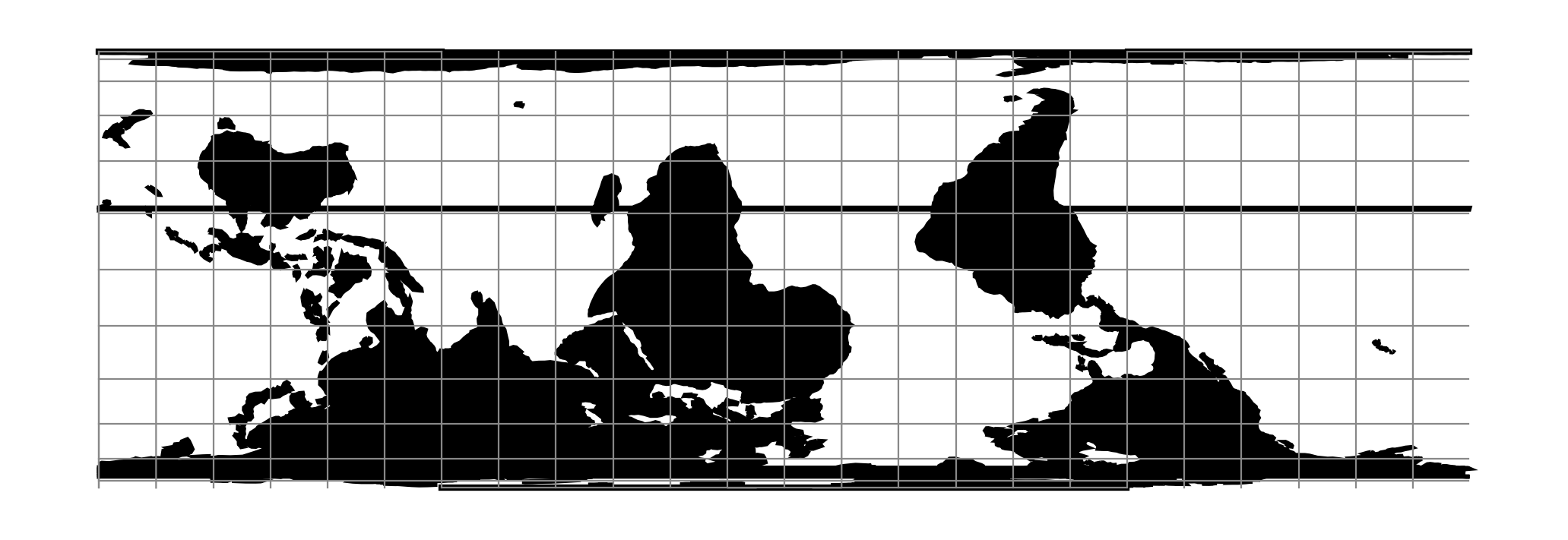# Oblique Cylindrical Equal Area¶proj-string: +proj=ocea

## Parameters¶

### Required¶

For the Oblique Cylindrical Equal Area projection a pole of rotation is needed. The pole can be defined in two ways: By a point and azimuth or by providing to points that make up the pole.

#### Point & azimuth¶

+lonc=<value>

Longitude of rotational pole point.

+alpha=<value>

Angle of rotational pole.

#### Two points¶

+lon_1=<value>

Longitude of first point.

+lat_1=<value>

Latitude of first point.

+lon_2=<value>

Longitude of second point.

+lat_2=<value>

Latitude of second point.

### Optional¶

+lon_0=<value>

Longitude of projection center.

Defaults to 0.0.

+R=<value>

Radius of the sphere given in meters. If used in conjunction with +ellps +R takes precedence.

+k_0=<value>

Scale factor. Determines scale factor used in the projection.

Defaults to 1.0.

+x_0=<value>

False easting.

Defaults to 0.0.

+y_0=<value>

False northing.

Defaults to 0.0.